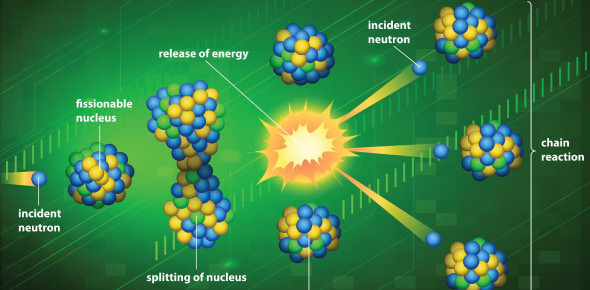# Atoms, Ions And Isotopes: Trivia Quiz!

11 Questions | Attempts: 1097Settings.

• 1.
What type of ion forms when an atom gains electrons?
• A.

Neutral

• B.

Positive

• C.

Negative

• D.

Possibly positive or negative

• 2.
What type of ion forms when an atom loses electrons?
• A.

Positive

• B.

Possibly positive or negative

• C.

Negative

• D.

Neutral

• 3.
Isotopes are atoms of the same element with the same number of protons and:
• A.

A different number of neutrons.

• B.

A different number of electrons.

• C.

A different number of molecules.

• D.

The same number of neutrons.

• 4.
Carbon-14 is a radioactive isotope used to determine the age of ancient objects composed of organic matter.  Carbon-12 and carbon-13 are other isotopes of carbon.  The number of subatomic particles varies among these carbon isotopes?
• A.

Electrons

• B.

Neutrons

• C.

Photons

• D.

Protons

• 5.
Lead (Pb-208) is the heaviest stable isotope known.  It has an atomic number of 82.  How many neutrons does Pb-208 have?
• A.

82

• B.

126

• C.

164

• D.

208

• 6.
The particular type of element is determined by the number of:
• A.

Electrons

• B.

Protons

• C.

Neutrons

• D.

Valence electrons

• E.

Energy levels

• 7.
An atom has six protons and eight neutrons.  Its atomic mass is ________________ atomic mass units.
• A.

Two

• B.

Four

• C.

Six

• D.

Eight

• E.

Fourteen

• 8.
Isotopes differ from each other with respect to the number of:
• A.

Protons only.

• B.

Neutrons only.

• C.

Electrons only.

• D.

Both protons and electrons.

• E.

Both neutrons and protons.

• 9.
The difference between an electrically neutral atom and an ion is that:
• A.

An ion has an unequal number of protons and electrons, while an atom has an equal number

• B.

An ion has an equal number of protons and electrons, while an atom has an unequal number

• C.

An atom has an unequal number of protons and neutrons, while an ion has an equal number

• D.

An atom has its electrons in orbitals, while an ion has its electrons in its nucleus

• E.

An atom must have an equal number of neutrons and electrons, while an ion does not.

• 10.
What is the maximum number of covalent bonds that can form between a single carbon and 1 or more hydrogen atoms?
• A.

1

• B.

2

• C.

3

• D.

4

• 11.
Breaking which type of bond would require the most energy?
• A.

Covalent

• B.

Electrostatic

• C.

Hydrogen

• D.

Intermolecular

## Related TopicsBack to top
×

Wait!
Here's an interesting quiz for you.